# Regression

## Regression

Given a table with point values (x, y) obtained from a mathematical model or from a physical process, the process of regression can be used to estimate any value of y given a value of x. A linear regression indicates that the variable y is a linear function of the variable x. If the scalar x is replaced by the vector x, then y is a linear combination of the values of x in the vector x.

## Regression in the output Layer

If the inverse of the activation function is applied to the target, it is possible to create a system of linear equations to find the weights in the output layer. This process is called regression. This system of equations may be solved by the method of Gauss Jordan; however, a better solution can be obtained using the method of Singular Value Decomposition.

## Singular Value Decomposition (SVD)

Basic linear algebra indicates that a matrix A where number of rows M is greater than or equal to the number of columns, can be written as the product of an orthogonal matrix U of size MxN, a diagonal matrix S with zero or positive elements, and the transposed of a orthogonal matrix V of size NxN as shown below.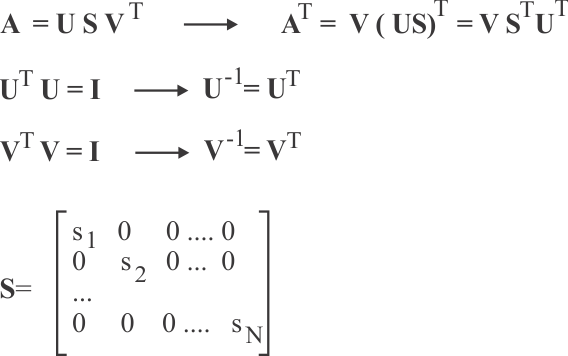Problem 1 Proof that the output weights of the ANN of the figure can be found as shown. Suppose that the activation function is z =tanh(ay).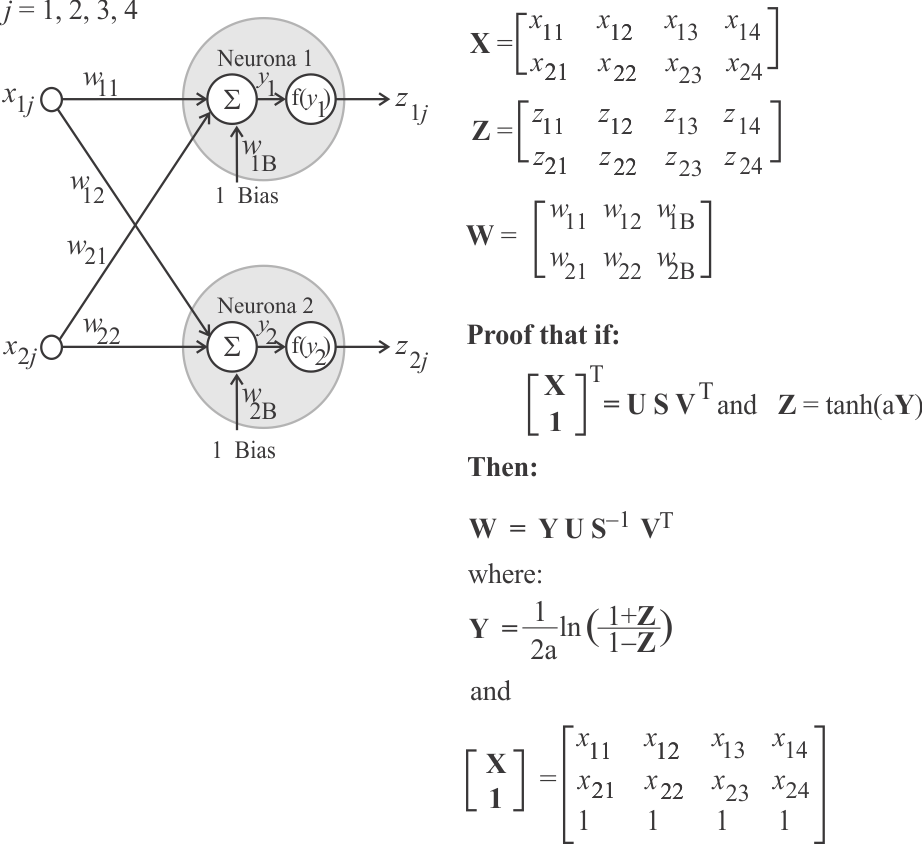Problem 2 Find the numeric values of Y in the neural network shown below when the activation function is z = logsig(y).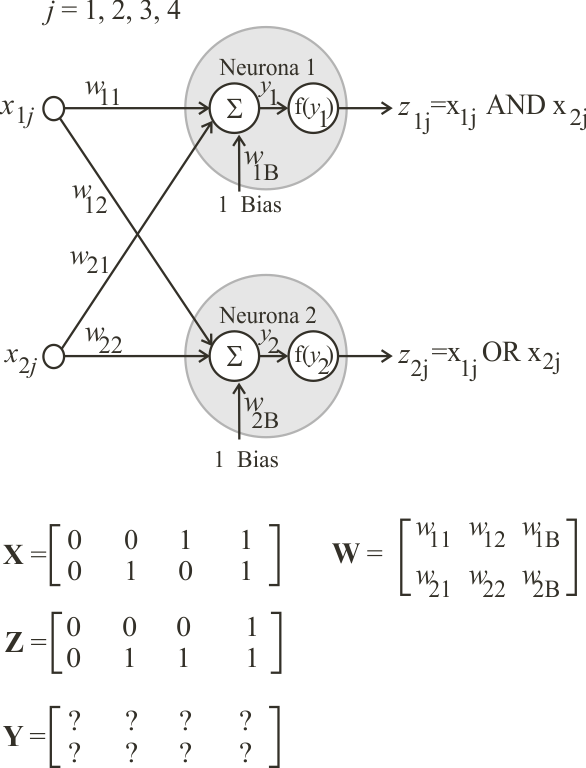Problem 3 Use Matlab to perform the Singular Value Decomposition of the matrix as shown below.

 solve.m A = [0, 0, 1;  0, 1, 1;  1, 0, 1;  1, 1, 1]; [U, S, V] = svd(A, 0);

 Problem 4 (a) Find the value of the weights of the output layer. As S is a diagonal matrix its inverse can be computed by finding the inverse of the elements in the diagonal. (b) Manually test the weights to verify that they produce the desired output.

 MSDOS: cmd.exe W =    20.0000   20.0000  -30.0000    20.0000   20.0000  -10.0000

 Problem 5 Create a Wintempla Dialog Application called SepProd using Microsoft Visual Studio to test Singular Value Decomposition.Cree una aplicación de diálogo de Wintempla llamada SepProd usando Microsoft Visual Studio para probar la Descomposición en Valores Singulares.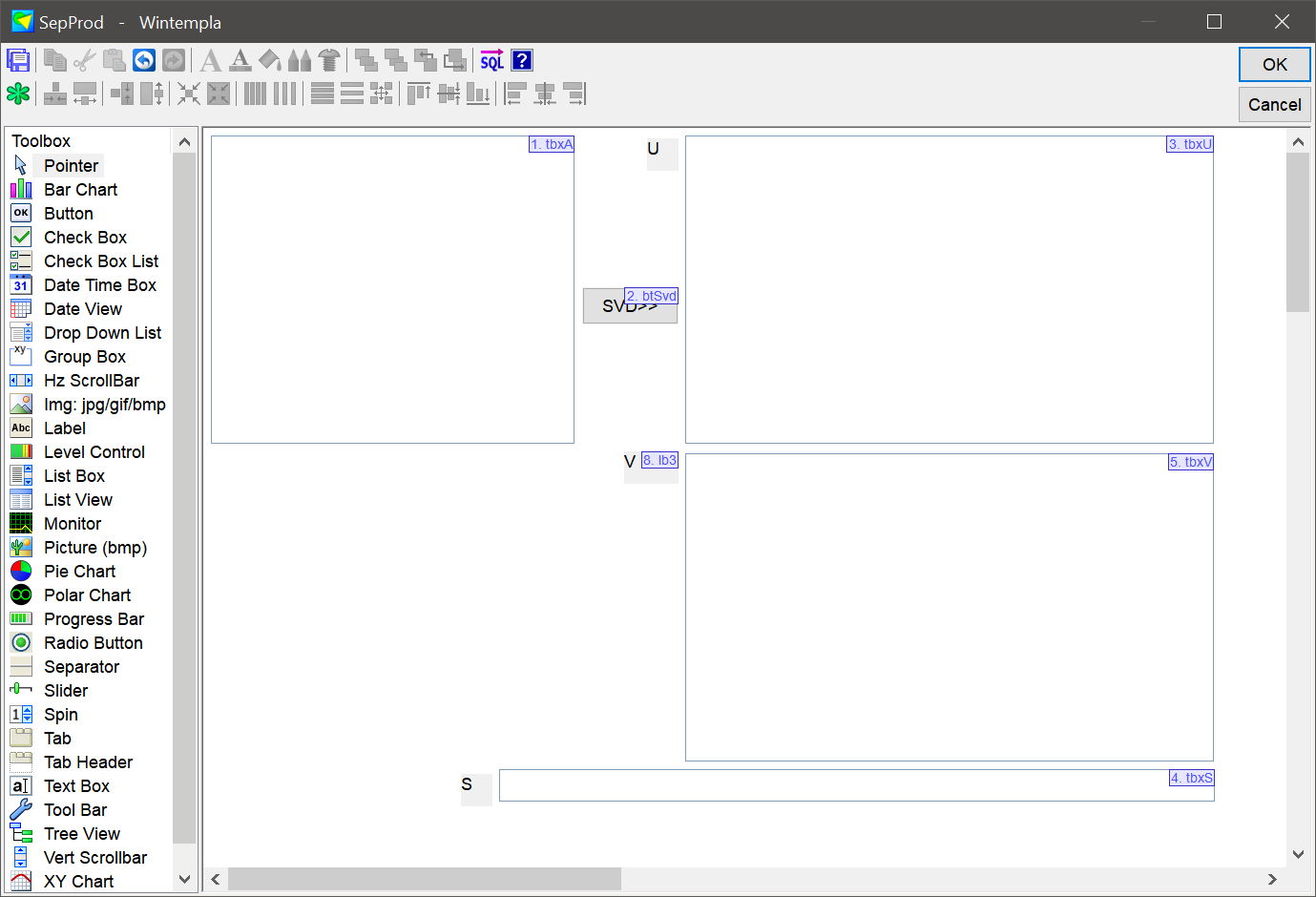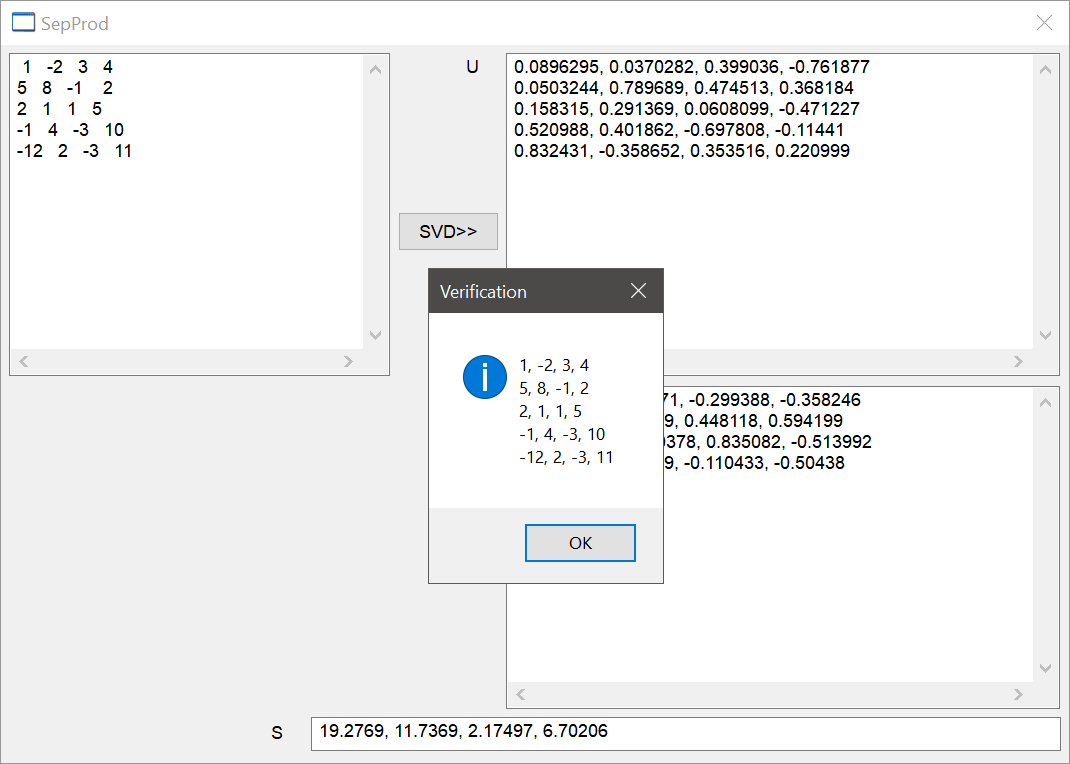SepProd.cpp ... void SepProd::Window_Open(Win::Event& e) { } void SepProd::btSvd_Click(Win::Event& e) {      //_________________________________________________ 1. Read A      MATRIX A;      Sys::Convert::ToMatrix(tbxA.Text, A);      //_________________________________________________ 2. SVD      MATRIX U, V;      valarray S;      const wchar_t* error = Math::SingValDecompos::Decompose(A, U, S, V);      if (error != NULL)      {           this->MessageBox(error, L"Math::SingValDecompos::Decompose", MB_OK | MB_ICONERROR);           return;      }      //_________________________________________________ 3. Show results      wstring text;      Sys::Convert::ToString(U, text);      tbxU.Text = text;      //      Sys::Convert::ToString(V, text);      tbxV.Text = text;      //      Sys::Convert::ToString(S, text);      tbxS.Text = text;      //_________________________________________________ 4. Verify results      const size_t rowCount = S.size();      MATRIX SM;      Math::Oper::CreateMatrix(SM, rowCount, rowCount);      for (size_t i = 0; i < rowCount; i++) SM[i][i] = S[i];      MATRIX result;      Math::Oper::Product(U, SM, result);      MATRIX VT;      Math::Oper::Transpose(V, VT);      Math::Oper::Product(result, VT, A);      Sys::Convert::ToString(A, text);      this->MessageBox(text, L"Verification", MB_OK | MB_ICONINFORMATION); }